## Iterate Subsystem Execution with While Iterator and For Iterator Subsystems

Iterator subsystems, such as the While Iterator and For Iterator subsystems, are a type of logically executed subsystem that executes one or more times during a time step in response to a control block internal to the subsystem block. The control block implements control logic similar to that expressed by a programming language construct such as a `while` loop or a `for` loop.

For information about the For Each subsystem, which partitions input signals or mask parameters into individual elements or subarrays and applies an algorithm to each, see For Each Subsystem.

Note

The While Iterator Subsystem and For Iterator Subsystem blocks must not contain blocks with continuous states (for example, blocks from the Continuous block library). The sample times of all blocks within the subsystem must be either inherited (`-1`) or constant `(inf)`.

### Models with While Structures

The While Iterator Subsystem block is a Subsystem block preconfigured as a starting point for creating a subsystem that repeats execution during a simulation time step while a logical (Boolean) expression is true.

Open the model `ex_while_iterator_block`.An input of `1` (`true`) to the While Iterator block activates the subsystem. At each time step, the current iterative number is added to a running total until a maximum sum is reached.

The while structure in the model can be represented with the following pseudo code.

```maximum_sum = 10 sum = 0 iteration_number = 1 condition = TRUE WHILE (condition is TRUE) AND (iteration_number <= maximum_iterations) sum = sum + iteration_number IF (sum > maximum_sum) THEN condition = FALSE END IF iteration_number = iteration_number + 1 END WHILE ```

#### Create Model with While Structure

To create the example model, use the following procedure.

1. Place a While Iterator Subsystem block in the Simulink® Editor. Double-click the subsystem block to display its contents.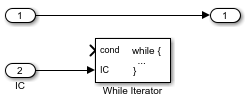2. Double-click the While Iterator block to open its block parameters dialog box. Set the Maximum number of iterations to `20` and States when starting to `reset`. Select the Show iteration number port check box.

3. Add Memory, Relational Operator, and Sum blocks. Connect blocks as shown. For the Memory block, select the Inherit sample time check box.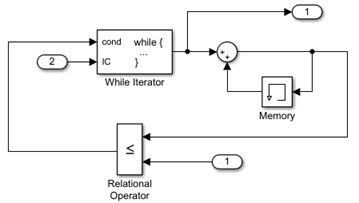The iteration number from the output of the While Iterator block is added to its previous value until the sum is greater or equal to the maximum sum from Inport block 1.

4. Navigate to the top level of the model.

5. Connect a Constant block to input port 2. This block provides the initial logical condition value for the While Iterator block. Set the Constant value to any non-zero number.

The While Iterator block requires an initial logical condition (input port labeled `IC`) for its first iteration. This signal enables the While Iterator Subsystem block and must originate from outside the subsystem. If this value is nonzero, the first iteration takes place.

6. Connect a second Constant block to input port 1. This block provides a maximum value for the iterative algorithm. The algorithm adds successive integers until a maximum value is reached.

7. Connect a Display block to output port 1. This block shows the number of iterations from the While Iterator block output port.

8. Run a simulation.

During a single time step, the first four iteration numbers are added for a total sum (`10`). With the fifth iteration, the sum (`15`) is greater than the maximum sum (`10`), the iterations stop, and the block waits for the next time step.

Note

Simulation time does not advance during iterative executions of a While Iterator Subsystem block. Nevertheless, blocks in the subsystem treat each iteration as a time step. As a result, in a While Iterator Subsystem block, the output of a block with states (that is, a block whose output depends on its previous input), reflects the value of its input at the previous iteration of the `while` loop. The output does not reflect the block input at the previous simulation time step. For example, a Unit Delay block in a While Iterator subsystem outputs the value of its input at the previous iteration of the `while` loop, not the value at the previous simulation time step.

### Model with For Structures

The For Iterator Subsystem block is a Subsystem block preconfigured as a starting point for creating a subsystem that repeats execution during a simulation time step for a specified number of iterations.

Open the model `ex_for_iterator_block`.The input to the For Iterator block specifies the number of iterations. At each time step, the current iterative number is added to a running total for `5` iterations.

The for structure in the model can be represented with the following pseudo code.

```number_of_iterations = 5 sum = 0 FOR iteration_number = 1 TO number_of_iterations sum = sum + iteration_number END FOR ```

#### Create Model With For Structure

To create the example model, use the following procedure.

1. Place a For Iterator Subsystem block in the Simulink Editor. Double-click the subsystem block to display its contents.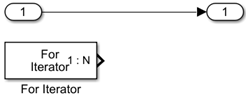2. Double-click the For Iterator block to open its block parameters dialog box. Set States when starting to `reset` and Iteration limit source to `external`.

3. Add Memory, Sum, and Outport blocks. Connect blocks as shown. For the Memory block, select the Inherit sample time check box.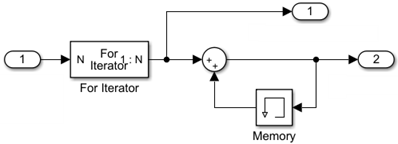The iteration number from the output of the For Iterator block is added to its previous value for the specified number of iterations from Inport block 1.

4. Navigate to the top level of the model.

5. Connect a Constant block to input port 1. This block provides the number of iterations for the For Iterator block. Set the Constant value to `5`.

6. Connect Display blocks to output ports 1 and 2. These blocks shows the number of iterations from the For Iterator block output port and the sum from the Memory block.

7. Run a simulation.

During each time step, the first five iteration numbers are added for a total sum (`15`).

#### Using Assignment Blocks

The For Iterator block works well with the Assignment block to reassign values in a vector or matrix. The following example shows the use of a For Iterator block. Note the matrix dimensions in the data being passed.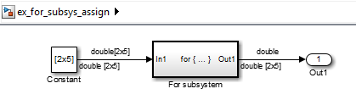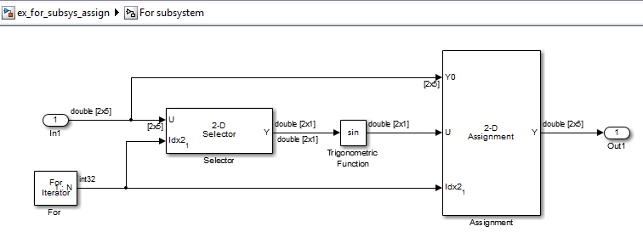The example outputs the sine value of an input 2-by-5 matrix (2 rows, 5 columns) using a For Iterator subsystem containing an Assignment block. The process is as follows.

• A 2-by-5 matrix is input to a Selector block and an Assignment block.

• The Selector block strips off a 2-by-1 matrix from the input matrix at the column value indicated by the current iteration value of the For Iterator block.

• The sine of the 2-by-1 matrix is taken.

• The sine value 2-by-1 matrix is passed to the Assignment block.

• The Assignment block, which takes the original 2-by-5 matrix as one of its inputs, assigns the 2-by-1 matrix back into the original matrix at the column location indicated by the iteration value.

Note that the Assignment block copies the first input (Y0) to the output (Y) only on the first iteration. The value of the second input (U) is copied to the output on the first and each subsequent iteration. All of these operations take place during a single time step.

The rows specified for reassignment in the parameter dialog box for the Assignment block in the example are [1,2]. Because there are only two rows in the original matrix, you could also have specified -1 for the rows (that is, all rows).

Note

The Trigonometric Function block is already capable of taking the sine of a matrix. The example uses the Trigonometric Function block only as an example for changing each element of a matrix with the collaboration of an Assignment block and a For Iterator block.